# Learn Arithmetic and Logical Operators in Python

1
5486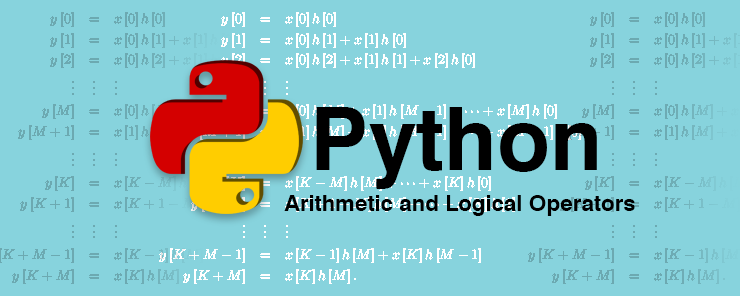After discussing the Variables and Basic Data Types in Part3 of Python Tutorial, it is time to know what to do with those variables. In other words, what operations can be performed using variables?

Arithmetic Operations in Python

One of the main reasons for writing computer programs is performing complex computations. All programming languages support mathematical operations using Arithmetic operators and functions.

The following table lists the Arithmetic operators Supported in Python:

 Operator Usage + Adds the two operands – Subtract the second operand from the first. * Multiply the first operand by the second one. / Divides the first operand by the second one. // Divides the first operand by the second one, and eliminates from the result the decimal point and any digits that appear on its right side. % Divides the first operand by the second one, and returns the reminder. ** The Power operator. It raises the first operand to the power of the second operand. +, – Plus and Minus signs. These are unary operators that take only one operand.

Example #1:

The following is a program that prompts the user to input three integers, and returns their summation and average.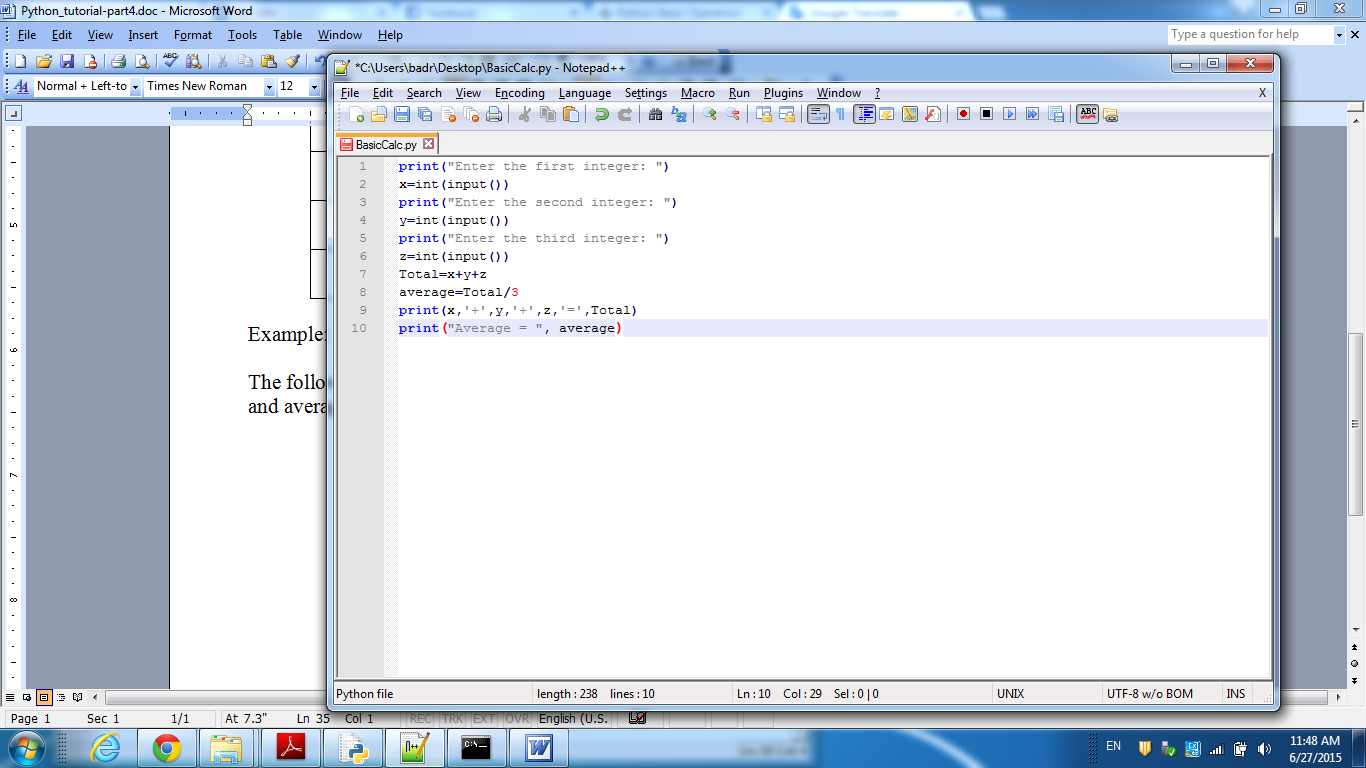Image 1: A Script that accepts three integers from the user and calculates their summation and average.

Let’s execute it and see the result: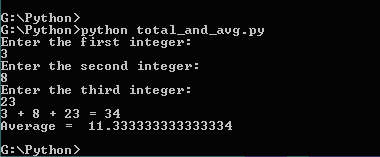Image 2: Executing the script that calculates the total and average.

Now, to the explanation:

Lines 1, 3, and 5: use the print() function to display the messages that ask the user to enter the first, second and third integers, respectively.

Lines 2, 4, and 6: use the input() function to wait for the user’s inputs. The function input() returns a string value that is passed as an argument to the function int(), to parse (convert) the entered string into integer.

Line 7: uses the “+” operator to add the three integers, then assigns the result to the variable Total.

Line 8: calculates the average of the three integers by dividing the Total by their count (3).

Lines 9, and 10: prints the calculated Total and average to the screen.

Arithmetic Assignment Operators:

A strange title, huh?!

– Mmm!! Yes, strange!! Is there assignment operators other than the equal sign “=” (which is the ordinary assignment operator)?!

Actually, yes, there are. Consider the following cases:

```Total = Total + 5

counter = counter + 1

loopvar = loopvar - 1

factorial = factorial * 6

half = half / 2```

There is one thing common in all of the statements above. The common thing is that a value is added, subtracted, multiplied, or divided into the variable on the left side of the operator, and the result of this operation is assigned to the same variable (the left-side variable). These are common operations that we need frequently to increment or decrement a counter, to calculate factorial, etc.

Python (and some other languages) have shorts for the above statements:

```Total += 5

counter += 1

loopvar -= 1

factorial *= 6

half /= 2
```

Aren’t they smart?!

Example:

In this example, we will rewrite the script in the previous example in a more elegant way.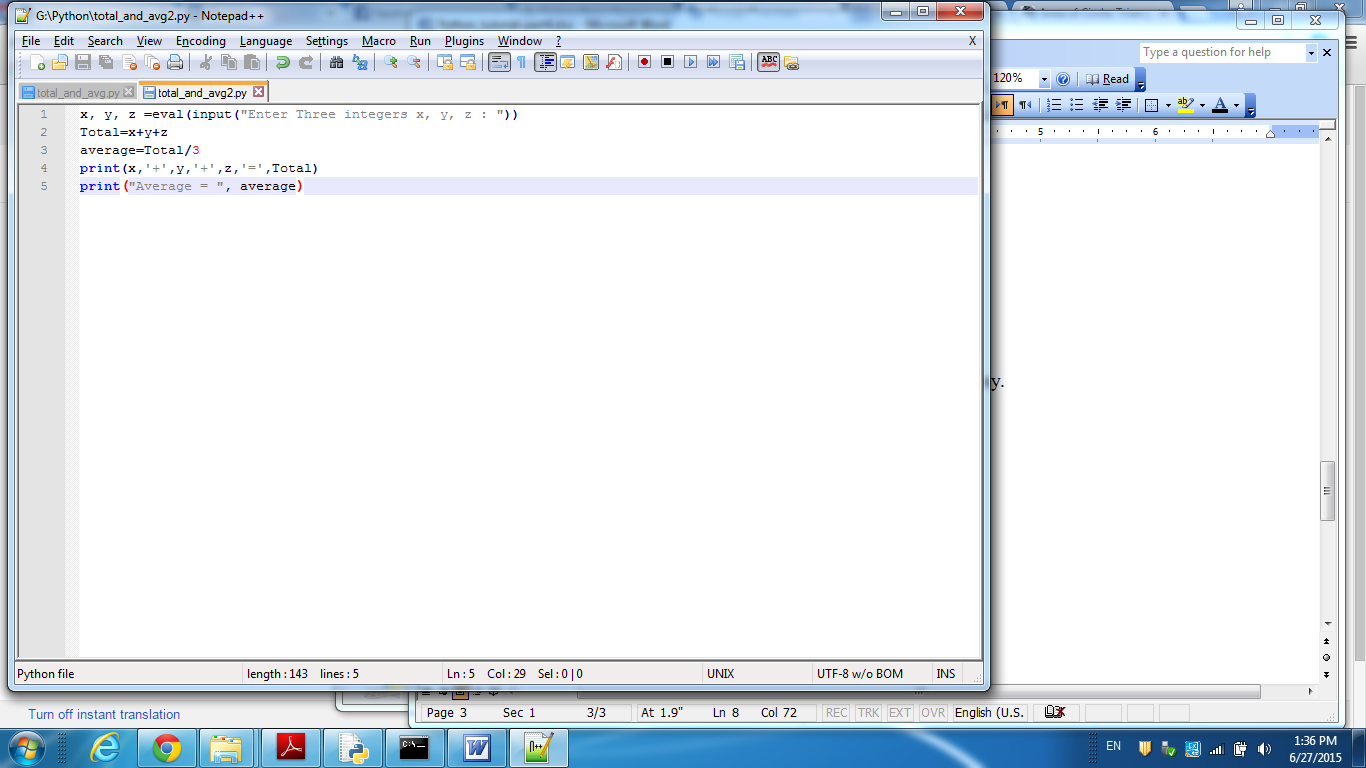Image 3: Rewriting the Total and Average Script.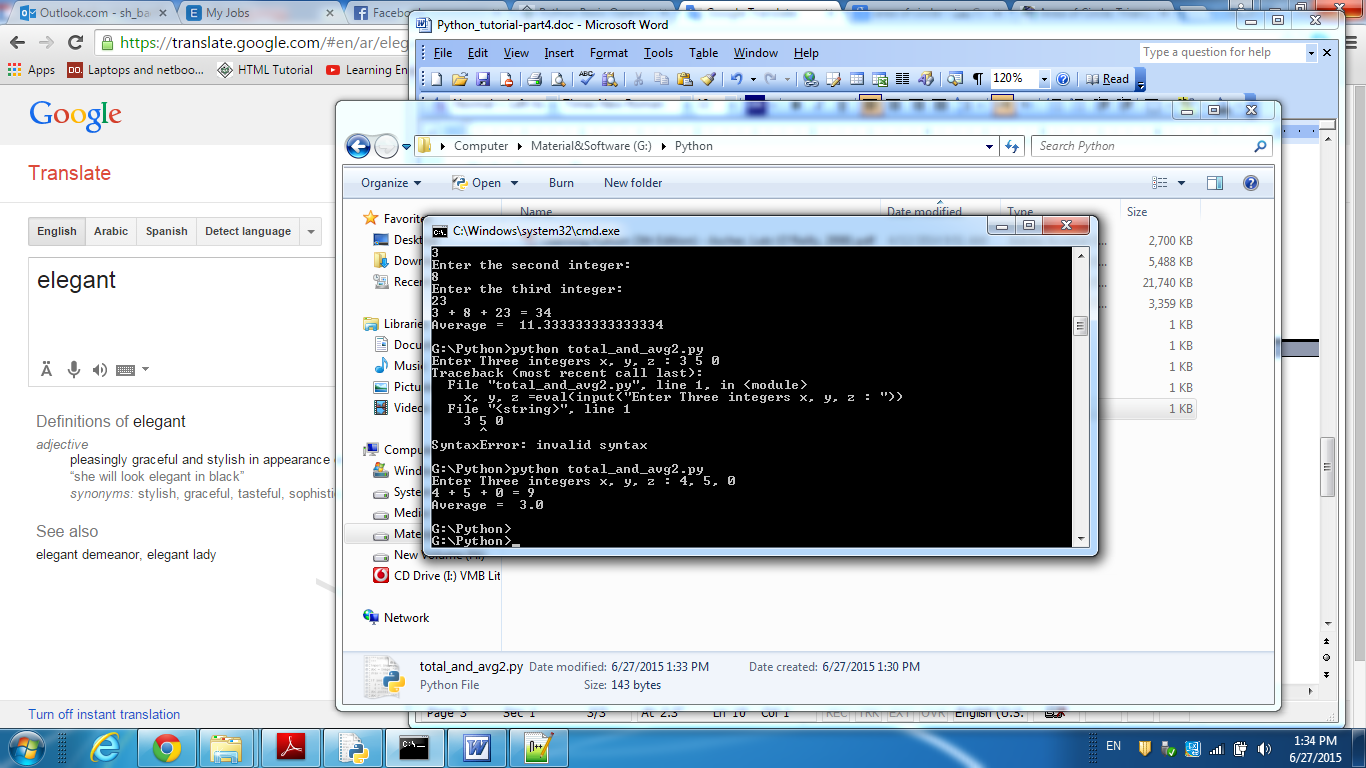Image 4: Executing the modified version.

Wow!! The number of lines in the script has dropped into half, while achieving the same result!! Interesting!!

Comparison and Logical Operators

The following table lists the Comparison operators with their usage. A Comparison operator is used to compare one operand to another, and returns either True or False. The True and False are called Boolean values.

 Operator Usage == Equality operator. Returns True if the two operands are equal. < Less than. Returns True if the left-side operand is less than the right-side operand. > Greater than. It is the opposite of the previous operator. <= Less than or Equal. Returns True if the left-side operand is less than or Equal to the right-side operand. >= Greater than or Equal. Returns True if the left-side operand is greater than or equal to the right-side operand. != Inequality Operator. Returns True if the two operands are not equal.

Let’s see them in action!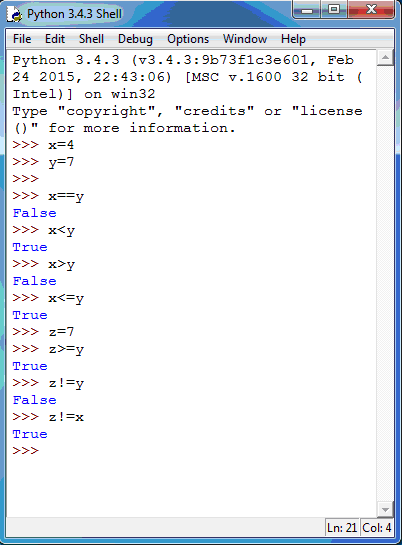Image 5: Some Examples to illustrate the Comparison Operators.

Okay, we have x=4, and y=z=7

Based on that:

1. The comparison to see whether x equals y (x==y) evaluates to False.
2. The comparison to see whether x is less than y (x<y) evaluates to True.
3. The comparison to see whether x is greater than y (x>y) evaluates to False.
4. The comparison to see whether x is less than or equal to y (x<=y) evaluates to True.
5. The comparison to see whether z is greater than or equal to y (z>=y) evaluates to True.
6. The comparison to see whether z is not equal to y (z!=y) evaluates to False.
7. The comparison to see whether z is not equal to x (z!=x) evaluates to True.

Logical Operators

What if we need to check whether x is less than y or not, and (at the same time) whether y is less than or equal to z? How could we achieve this requirement?

Logical Operators are the solution.

There are three basic Logical Operators: AND, OR, and NOT. When we say basic, you could understand that there are other operators that are considered to be composite (like NAND, NOR, XOR, and XNOR). Such composite operators are derivatives of the basic operators, and are results of their interactions. In this section, we will talk about the three basic logical operators.

AND

Consider an electrical circuit that has two switches connected in series. For the current to flow from one side to the other through the switches, both switches must be ON. In other words, Switch 1 should be ON, AND Switch 2 should be ON, for the current to travel and the result to be ON.

Truth Table for the Logical AND operation:

 Switch1 Switch2 Result Off Off Off Off On Off On Off Off On On On

OR

Now, consider the switches are connected in parallel. For the current to flow from one side to the other, only one of the switches needs to be ON. In other words, if switch 1 is ON OR Switch 2 is On, the result will be ON.

Truth Table for the Logical OR operation:

 Switch1 Switch2 Result Off Off Off Off On On On Off On On On On

NOT

The NOT operator acts like an inverter, that inverts the status of its input. The Truth Table for the NOT operation:

 Input Output Off On On Off

Now, let’s illustrate the theoretical concepts by examples: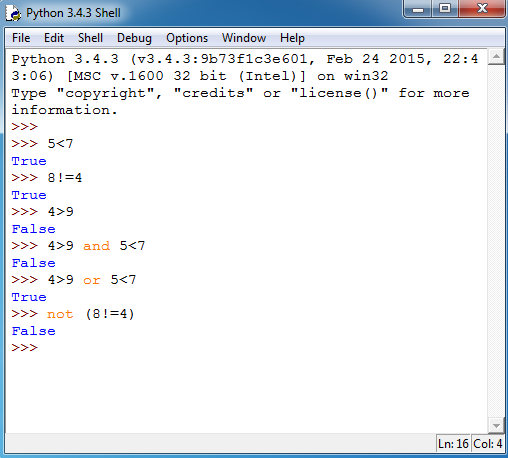Image 6: Examples for Logical Operators.

Got the idea?! Great!!

Now, you are ready for the Next topic: Decision making using IF Conditions

So, don’t miss it!!

#### 1 COMMENT

1.Meenakshi Agarwal

Very useful. It is nicely addressing all the arithmetic operations one can perform using Python data types. Thanks.